# 构建卷积并执行池化

• 什么是卷积
• 如何创建特征图
• 什么是池化

• 图像的特征图

## 2. 什么是卷积？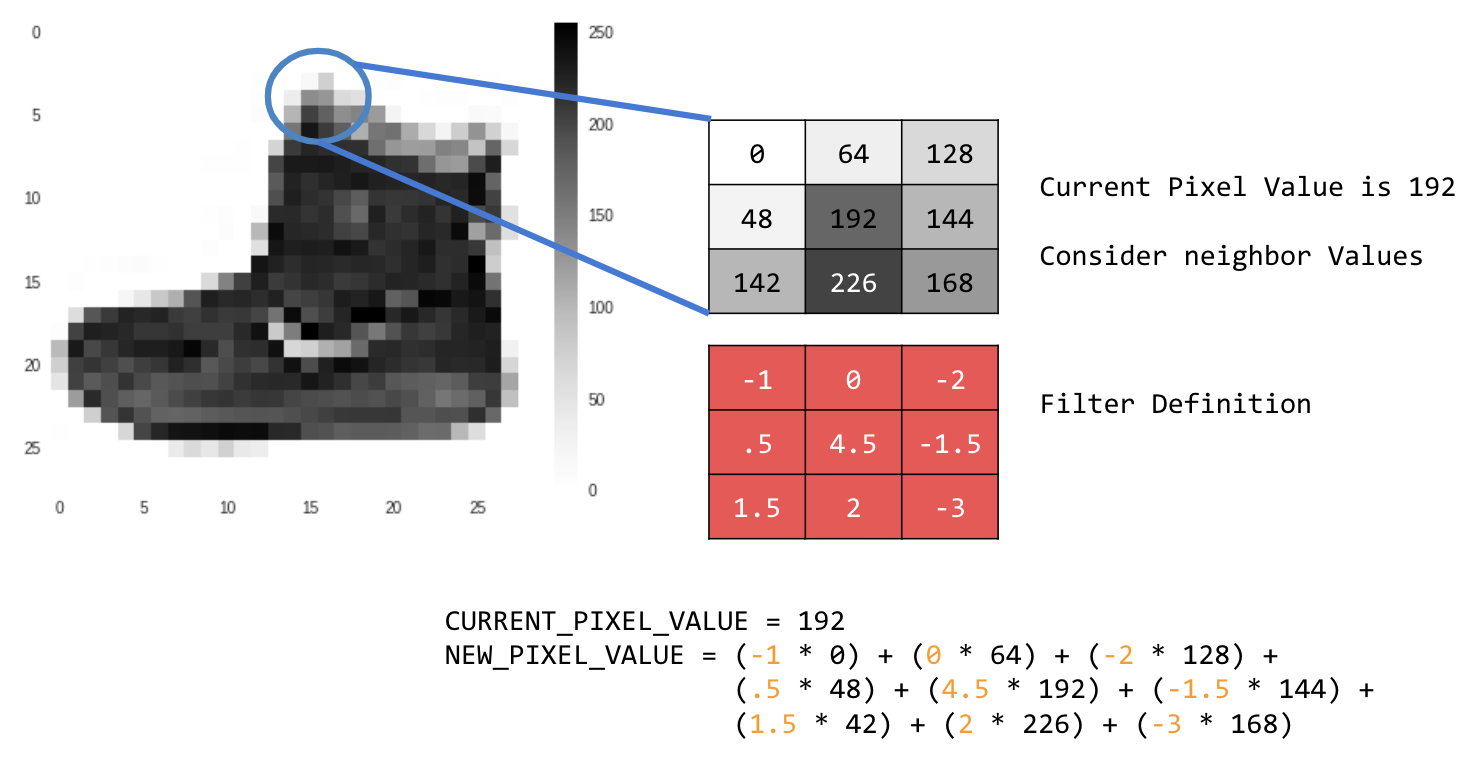## 3. 开始编码

``````import cv2
import numpy as np
from scipy import misc
i = misc.ascent()
``````

``````import matplotlib.pyplot as plt
plt.grid(False)
plt.gray()
plt.axis('off')
plt.imshow(i)
plt.show()
``````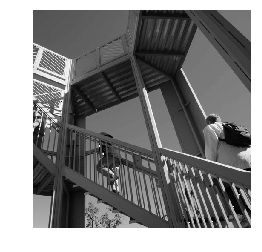``````i_transformed = np.copy(i)
size_x = i_transformed.shape
size_y = i_transformed.shape
``````

## 4. 创建卷积矩阵

``````# This filter detects edges nicely
# It creates a filter that only passes through sharp edges and straight lines.
# Experiment with different values for fun effects.
#filter = [ [0, 1, 0], [1, -4, 1], [0, 1, 0]]
# A couple more filters to try for fun!
filter = [ [-1, -2, -1], [0, 0, 0], [1, 2, 1]]
#filter = [ [-1, 0, 1], [-2, 0, 2], [-1, 0, 1]]
# If all the digits in the filter don't add up to 0 or 1, you
# should probably do a weight to get it to do so
# so, for example, if your weights are 1,1,1 1,2,1 1,1,1
# They add up to 10, so you would set a weight of .1 if you want to normalize them
weight  = 1
``````

``````for x in range(1,size_x-1):
for y in range(1,size_y-1):
output_pixel = 0.0
output_pixel = output_pixel + (i[x - 1, y-1] * filter)
output_pixel = output_pixel + (i[x, y-1] * filter)
output_pixel = output_pixel + (i[x + 1, y-1] * filter)
output_pixel = output_pixel + (i[x-1, y] * filter)
output_pixel = output_pixel + (i[x, y] * filter)
output_pixel = output_pixel + (i[x+1, y] * filter)
output_pixel = output_pixel + (i[x-1, y+1] * filter)
output_pixel = output_pixel + (i[x, y+1] * filter)
output_pixel = output_pixel + (i[x+1, y+1] * filter)
output_pixel = output_pixel * weight
if(output_pixel<0):
output_pixel=0
if(output_pixel>255):
output_pixel=255
i_transformed[x, y] = output_pixel
``````

## 5. 查看返回的结果

``````# Plot the image. Note the size of the axes -- they are 512 by 512
plt.gray()
plt.grid(False)
plt.imshow(i_transformed)
#plt.axis('off')
plt.show()
``````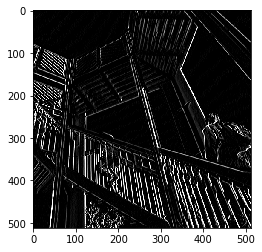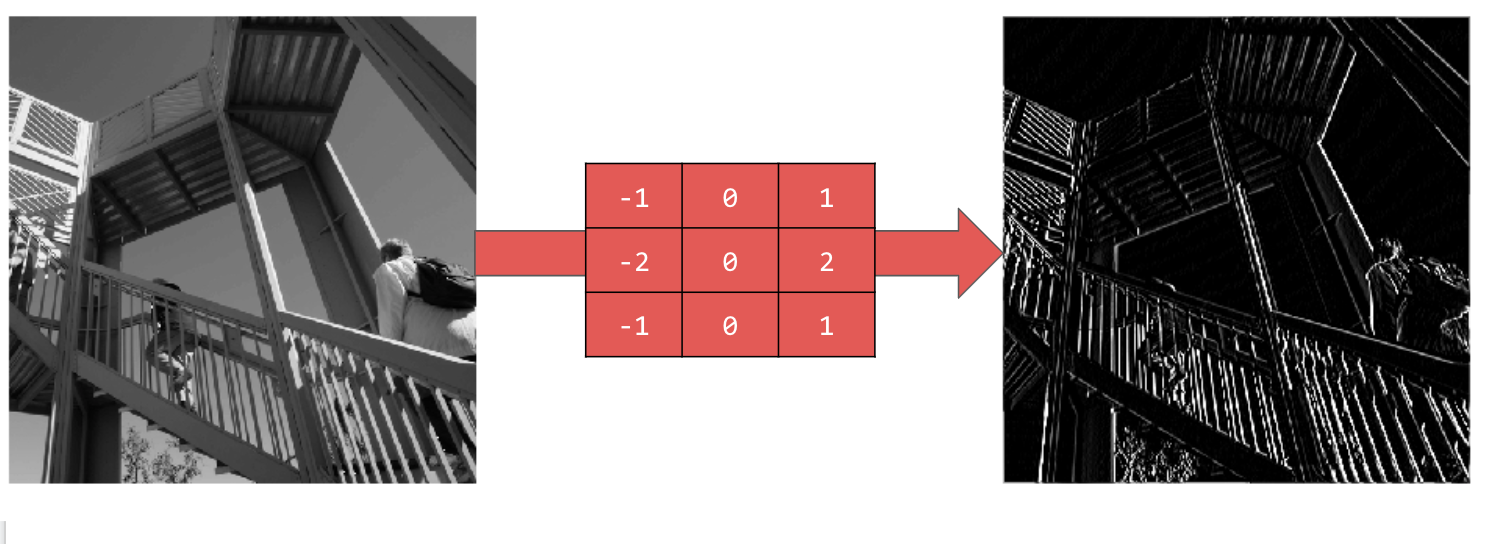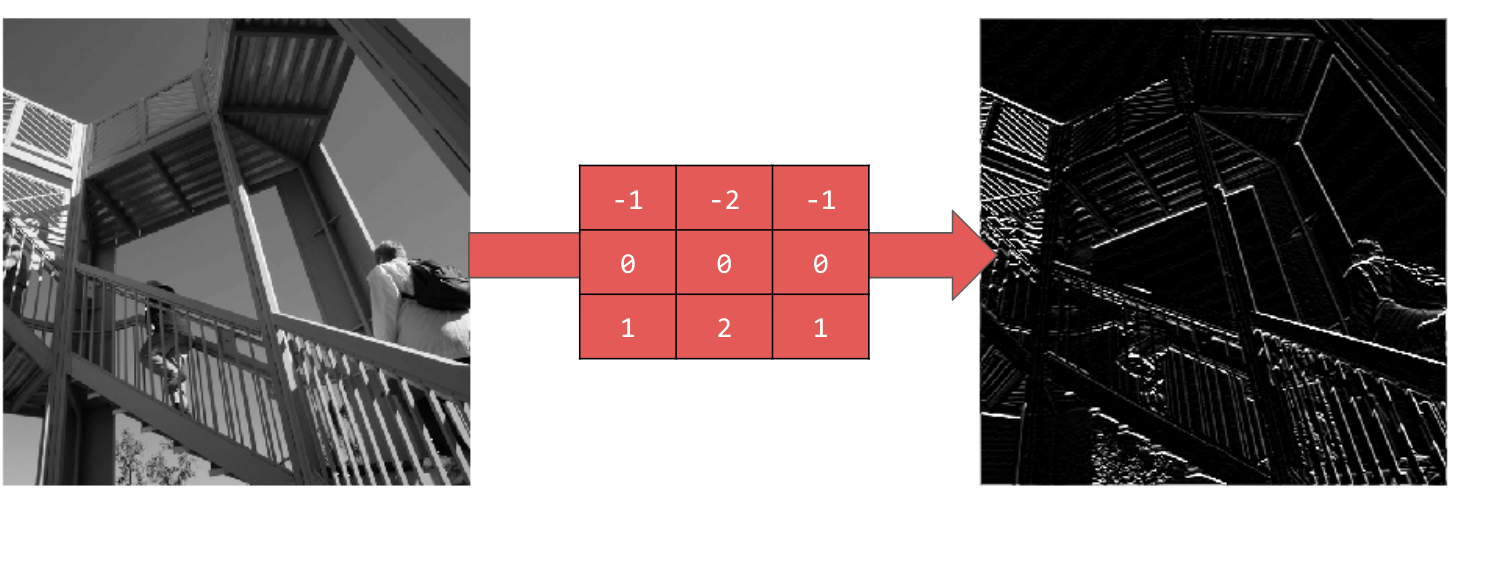## 7. 编写池化代码

``````new_x = int(size_x/2)
new_y = int(size_y/2)
newImage = np.zeros((new_x, new_y))
for x in range(0, size_x, 2):
for y in range(0, size_y, 2):
pixels = []
pixels.append(i_transformed[x, y])
pixels.append(i_transformed[x+1, y])
pixels.append(i_transformed[x, y+1])
pixels.append(i_transformed[x+1, y+1])
pixels.sort(reverse=True)
newImage[int(x/2),int(y/2)] = pixels

# Plot the image. Note the size of the axes -- now 256 pixels instead of 512
plt.gray()
plt.grid(False)
plt.imshow(newImage)
#plt.axis('off')
plt.show()
``````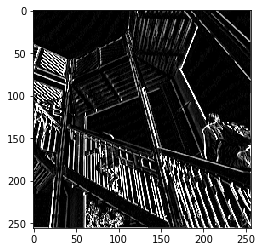## 8. 恭喜

[{ "type": "thumb-down", "id": "missingTheInformationINeed", "label":"没有我需要的信息" },{ "type": "thumb-down", "id": "tooComplicatedTooManySteps", "label":"太复杂/步骤太多" },{ "type": "thumb-down", "id": "outOfDate", "label":"内容需要更新" },{ "type": "thumb-down", "id": "translationIssue", "label":"翻译问题" },{ "type": "thumb-down", "id": "samplesCodeIssue", "label":"示例/代码问题" },{ "type": "thumb-down", "id": "otherDown", "label":"其他" }]
[{ "type": "thumb-up", "id": "easyToUnderstand", "label":"易于理解" },{ "type": "thumb-up", "id": "solvedMyProblem", "label":"解决了我的问题" },{ "type": "thumb-up", "id": "otherUp", "label":"其他" }]